﻿ Darcy’s Law Equation with Application to Underground Seepage in Earth Dams in Calculation of the Amount of Seepage

### Darcy’s Law Equation with Application to Underground Seepage in Earth Dams in Calculation of the Amo...

Koros Kiplangat Vincent, Mutua Nicholas Muthama, Sakayo Nicodemus MuokiOPEN ACCESSPEER-REVIEWED

## Darcy’s Law Equation with Application to Underground Seepage in Earth Dams in Calculation of the Amount of Seepage

Koros Kiplangat Vincent1, Mutua Nicholas Muthama1,, Sakayo Nicodemus Muoki1

1Mathematics and Informatics Department, Taita Taveta University College, Voi, Kenya

### Abstract

Water is a very important natural resource that no creation can exist without it. Hence it has become a responsibility of every person to try and conserve this very precious resource. One of the ways of conservation is by building earth dams. However if they are not well managed these dams may end up drying up due to underground seepage leading to failure of the dam. In this paper we are concerned with how to calculate the discharge rate through a porous medium, the velocity of the discharge and finally the total discharge using Darcy’s law. This study will also describe a computer based model that accepts input and calculate the amount of discharge per given time. From Darcy’s law it is shown that, the discharge rate depends among other factors the porosity of the medium. So from the study we were able to determine which medium is able to hold water rather than supporting percolation.

### At a glance: Figures

123
Prev Next

• Vincent, Koros Kiplangat, Mutua Nicholas Muthama, and Sakayo Nicodemus Muoki. "Darcy’s Law Equation with Application to Underground Seepage in Earth Dams in Calculation of the Amount of Seepage." American Journal of Applied Mathematics and Statistics 2.3 (2014): 143-149.
• Vincent, K. K. , Muthama, M. N. , & Muoki, S. N. (2014). Darcy’s Law Equation with Application to Underground Seepage in Earth Dams in Calculation of the Amount of Seepage. American Journal of Applied Mathematics and Statistics, 2(3), 143-149.
• Vincent, Koros Kiplangat, Mutua Nicholas Muthama, and Sakayo Nicodemus Muoki. "Darcy’s Law Equation with Application to Underground Seepage in Earth Dams in Calculation of the Amount of Seepage." American Journal of Applied Mathematics and Statistics 2, no. 3 (2014): 143-149.

 Import into BibTeX Import into EndNote Import into RefMan Import into RefWorks

### 1. Introduction

Darcy’s law is a simple proportional relationship between instantaneous discharge rate through a porous medium, the viscosity of the fluid and the pressure drop over a given distance. It forms the scientific basis of fluid permeability used in earth sciences particularly in hydrogeology. It is based on the flow of water through beds of sand. An application of Darcy’s law is to water flow through an aquifer. We can apply this law to the water flow under the bed of a dam; Darcy’s law along with the equation of conservation of mass is equal to the ground water flow equation.

Darcy’s law is valid for laminar flow in pores of a filter medium and seepage is normally a laminar flow in most cases, for example in sandy and clay soils. So in this case we are going to consider the case of a laminar flow. A laminar flow is sometimes referred to as the streamline flow. It occurs when a fluid flows in parallel layers with no disruption between the layers. There are no cross currents perpendicular to the direction of flow, no eddies or swirls of fluids. Laminar flow occurs at low Reynolds numbers where viscous forces are dominant and is characterized by smooth constant fluid motion.

### 2. Literature Review

The first contributor to underground water movement was  who studied the determination of laws of flow of water through sand. Darcy conducted experiments relating to water’s flow through sand, which resulted in the development of Darcy’s Law.  published the law in 1856 in a report, Les fontainespubliques de la ville de Dijon.  discovered that the flow through the pipe is proportional to the head differential, and is also proportional to a coefficient related to the nature of the sand. This coefficient is what we now understand as hydraulic conductivity.

 described how to prepare an upstream flow of a dam; Remove the pervious and decaying matter by breaking up the natural soils and by stepping up the sides of the ravine. One of his axioms is that water abhors an angle. The stepping toothed trenches need not necessarily be made by means of inclined and horizontal planes but should be wedge shaped so that the pressure of fluid in settling will wedge the material tighter together to fill every cavity.

 states that the rate of infiltration of soil depends upon its porosity which governs the functional to flow, and the slope and length of the filamentary channels along which the water may be considered to pass. It is therefore evident that the direct rate of infiltration in a homogeneous soil must decrease from the top to the bottom of the puddle trench. The best section for a puddle trench is thus a wedge, such as an open exacation.

 tried to calculate what height of dam is recommended for a given volume of water the dam should hold.  argued that the height of the dam depends with the storage required in the reservoir. For construction materials he suggested that; the materials should preferably be taken from the reservoir site, different parts of the sides of the valley should be examined so that the most suitable soils are located. However some water will seep through the dam, even if it is constructed of good materials, and well-compacted. This seepage reduces the strength of the dam.  recommends the crest width and slopes to provide a stable, 3m-h embankment making extra seepage protection unnecessary. A safer, but technically difficult, solution is to include a rock to drain, to collect seepage water. This should extend up to a third of the height of the dam, and a graded sand and gravel filter must be placed between the dam fill material and the drain to prevent fine clay particles being washed out. The filter must be designed according to the particle size of the dam material and the drain, following, for example, recommendations in Schwab et al, p488-490.

Darcy´s filtration law and continuity equation are applied to the solution of seepage by classical methods. For the calculation of the seepage through the body of the Starina dam, Myslivec’s relation  for a heterogeneous dam with a vertical centric seal was applied. In order to calculate seepage through the subsoil, a derived relation by  for subsoil bearing a vertical sealing element was applied. The total seepage through the body and subsoil of the Starina dam determined by a classical calculation method is 4.33 l.s-1.

 studied detection of seepage paths in earth dams using self-potential and electrical resistivity methods. A case of two of the four Saddle dams of the Som-Kamla-Amba project, Rajasthan State, India; which is founded on heterogeneous rock mass was taken. Electrical resistivity method was used to delineate zones favorable for seepage, whereas, self-potential (SP) method was used to delineate the seepage paths. SP measurements showed negative anomaly of the order of 10–20 mV in amplitude, indicating low seepage, coinciding with the seepage measurements made by the project authorities.  presented a practical finite difference method for unconfined seepage, which can be easily implemented in spreadsheets. The finite difference equations are based on the concepts of extended pressure and flux conservation. The proposed method eliminates the formation of matrix systems at the expenses of slower convergence rate for large problems. It has not only educational but also practical values as it applies to various engineering problems. A spreadsheet application of three-dimensional seepage modeling with an unknown free surface has been carried out. One of the main advantages of this study is that only one finite difference equation has been applied to the solution domain instead of derivation of additional finite difference equation to impervious boundary conditions, inclined interfaces, etc.

 six experiments were done in the laboratory of GeoDelt to investigate the possibility and effectiveness of Biosealing for seepage prevention, four with nutrition injection and two without, serving as contrast experiments. Biosealing refers to a natural biological process for self-detection and sealing of seepage in water impermeable barriers. BioSealing is based on the principle that biological activity can be stimulated by injection of nutrition in the soil. Analyses of the experimental results show that BioSealing is an effective way to reduce the seepage discharge and to decrease the soil permeability.

 conducted non destructive evaluation of seepage. During the summer of 2007 seepage was identified at the foot of the dam at high reservoir conditions. After long droughts, 2010 proofed to be a wet year and the reservoir levels rose to where it was in 2007. Seepage was observed about 30 feet downstream from where it was in 2007. The Willowstick was contracted to perform a geophysical investigation of seepage through the Vermilion Valley dam. The results of their investigation were presented, as well as proposed upgrades to the filter system at Vermilion Valley Dam. While the Willowstick survey did not directly identify the source of the boil, it did identify seepage paths that likely intercept the herringbone drain and thus provide the source for the seeping boil. This information will be important in the drain improvement program that continues at Vermilion Valley Dam. In the case of Vermilion Valley Dam, even though the seepage information did not lead directly to the source of the seepage, the information obtained from the Willowstick survey yielded insight into conditions for which the drains were installed and whether these drains need to be modified or augmented in order to allow continued safe and efficient operation of the dam.

For simulation  used software based on the finite elements technique that is able to simulate and analyze isometric distribution water through soils and rocks. They used the software (seep/w) to simulate and investigate seepage through dams. Continuity phase of the liquid, Darcy equation behavior of seep zone and UN isotropic are the assumptions utilized in the equations. In a porous environment analysis with different boundary conditions effectively been used. In computational program two dimensional analyses were successfully carried out with the assumption of uniform seepage at critical section.  recorded different hydraulic gradient coefficients by the flow rates at different layers of the dam.

2.1. Statement of the Problem

When a dam is constructed on a porous medium where the underlying soil is unsaturated the water may percolate into medium due to mounting pressure by the water behind the dam. Due to pressure difference (gradient) at different points of the fluid path in the medium, the fluid will flow leading to drainage of water. We therefore intend to calculate the flow rate of water along the path, the flow velocity and the total discharge. We need to study the porosity of different soils and pressure drop over a given distance along the fluid path.

2.2. Objectives of the Study

The objectives of the study are;

i) To determine the total seepage of water through a porous medium and compare the same through different materials.

ii) To determine the pore velocity of the fluid through the medium.

2.3. Application of Study

The study of seepage in earth dams is a very important consideration as far as the environment around us is concerned. It is because it assists in minimizing loss of water in the dam especially in arid and semi arid areas where the there is no regular inflow to the dam. It will also assist in controlling the removal and carrying away of soil particles (soil erosion). Excessive seepage pressure or soil saturation can threaten the stability of the downstream slope of the dam or the abutment slopes and hence water reservoir can collapse any time leading to total loss. Darcy’s law application is to water flow through an aquifer and it is also used extensively in petroleum engineering to determine the flow through permeable media the most simple of which is for one dimensional homogeneous rock formation with a fluid of constant viscosity.

2.4. Equations governing the Flow

In this section the equations governing hydrodynamics and more specifically underground fluid flow are outlined. Also in the chapter are the assumptions made during the course of the study and consequences arising from these assumptions. The equation of continuity, momentum and energy are stated together with their derivation. Darcy’s law equation which describes the flow through a porous media is also stated and derived followed by a model description of seepage under a dam. We will also derive underground water flow equation from equation of continuity and application on Darcy’s law. We will then using Crank Nicholson numerical method which uses finite differences generate a tri-diagonal matrix. The underground water flow equation is some sort of diffusion equation. Lastly we apply the Wolfram Mathematica software to solve the generated matrix.

2.4.1. Assumptions and Approximations

The following assumptions have been made;

i. The fluid flow under consideration is a laminar flow.

ii. The fluid is incompressible (the change in density is negligible).

iii. The medium through which the seepage of the fluid is taking place is porous.

iv. The fluid is inviscid (fluid not viscous).

v. The flow is in the x-direction only.

2.4.2. Consequences as a Result of the Assumptions

We have made an assumption that the fluid under considerations is incompressible but in the real sense it is not because of the difference in temperature in different parts of the porous material. However the change is very insignificant that it will bring very small changes to our calculations.

We have also assumed the seepage is only in one direction. In reality the flow of water or any fluid might not be in the x direction only. Some of the fluid particles will take the y and even the z directions. This will bring some errors to the final result of total seepage as the flow is only in one dimension.

2.5. The Governing Equations

There are three equations that govern the flow, namely;

Equation of conservation of mass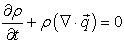(1)

Equation (1) is the general equation of conservation of mass of a fluid flow whose velocity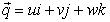. If the fluid is incompressible and the fluid flow is steady equation (1) becomes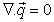Equation of energy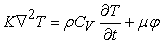(2)

Equation (2) is the equation of energy of a non- viscous incompressible fluid with constant coefficient of conductivity.

Ground water flow equation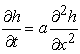(3)

This is the 1D underground water flow equation which resembles 1D heat equation (diffusion equation). We can say that this is a diffusion equation as water move with a very slow velocity from a region of high to low concentration.

2.6. Numerical Solution
2.6.1. The Crank Nicolson Method

We have applied the Crank Nicolson numerical method to solve arising equation. It is a finite difference method used for numerically solving heat equation and similar partial differential equations like in our case here the 1D ground water flow equation. It is a second order method in time, implicit in time and is numerically stable. It solves both the accuracy in stability problem. The difference equation for the ground water flow equation is;

Let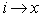and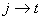.

Thus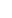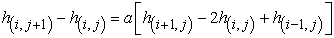.

Or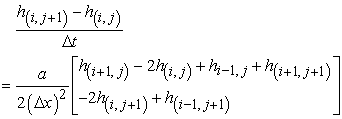.

Or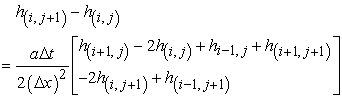.

Let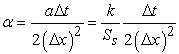.

Therefore we get;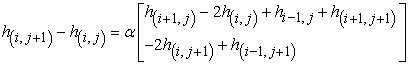Putting the terms containing j+1 on the left hand side and putting the others on the right hand side we get;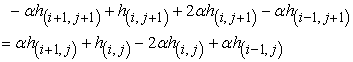And collecting the like terms together we come up with the following equation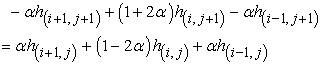The following graph shows how the scheme works

The equation above can be rewritten as a set of simultaneous equations which generates a tridiagonal matrix as shown below2.6.1. Solving the Tridiagonal Matrix

The solution to above triadiagonal matrix gives us the value of ‘h’ at different values of ‘b’ and ‘α’. Let the simultaneous equations be written as difference equation. And in the meantime time let –α=a=c, and 1+2α=b, then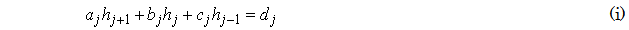Introducing new unknowns ej and fj along with an equation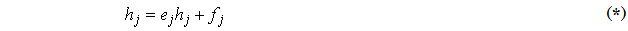And writing with shifted index we get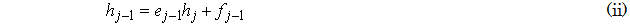And substituting for the value of hj-1in equation (i) we get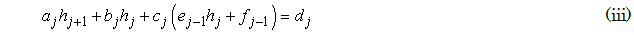Then rearranging to make hj the subject of the formulas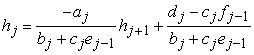Comparing this equation with equation (*) we simply express the introduced unknowns in terms of a, b and c.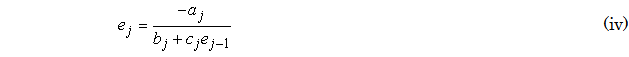2.6.2. Boundary Conditions

In order to find a solution to this problem we must have the boundary conditions. First, the boundary condition for the left hand side must be given. The most general possible end condition is a linear relation given as;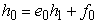Which is similar to the equation (*) above. This boundary condition must give us both e0 and f0 with e0 and all aj, bj and cj we can use equation (iv) to compute all the ej.

Similarly the right hand boundary condition can be given as a general two point boundary condition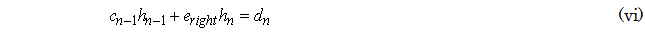This include a special case the zero value and the zero slope boundary condition. Equation (vi) can be compared to equation (iii).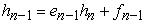You realize that both hn and hn-1 are unknowns but in equations (vi) and (vii) we have two equations. The first step is to take the value of hn and used it in equation (iii) to solve for hn-1, hn-2, hn-3 etc.

2.6.3. Equations Used in Calculation

The main objective of this study is to calculate the velocity of the seepage and finally the amount of seepage at certain time and distance. The following are our guiding equations.Which is the underground water flow equation in one dimension. Where;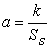And K is the permeability of the porous media and Ss is the specific storage or actually the amount of water that is available for seepage.Where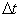is the change in time and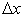is the change in distance in the x direction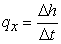the mass flux

And the pore velocity is given by the relation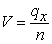where n is the porosity of the porous material.

Solution to the above equations will give us the value of α which will be used in solving the matrix. The solution to the matrix gives the value of total seepage in the x-direction given by h.

2.6.4. Underground Water Flow Equation Solution by MATLAB

This code in MATLAB uses the above equations to find the value of α which is used in the matrix to solve for total seepage and the seepage velocity at any one point. The seepage calculation is only in the positive x-direction.

//Matlab code that solve underground water flow equation

//via the Crank Nicholson scheme

//Developed by Vincent Koros during the month of March 2012

//dt=change in time,dx=change in distance in the positive x direction

//Ss=specific storage,k=permeability,n=porosity

//'b','c' and 'd' are the terms of the matrix

if(dx<=0|dt<0)

'Either dx or dt NOT a valid Value.Input it again!!!'

else

//initialise the specific storage to 200

ss=200;

//solve for 'a' which is later used to solve for alpha

a=k/ss; alpha=(a*dt)/(2*dx^2);

//iniatialise the terms of the matrix and develop the matrix A

b=1+2*alpha; c=-alpha;d=c;

A=[b,c,0,0,0;c,b,d,0,0;0,c,b,d,0;0,0,c,b,d;0,0,0,c,b;];

//calculate a constant 'm' which is used to iniatialise the right hand vector

m=c/b;

w=10; x=w+m*w; y=x+m*x; z=y+y*m;r=z+z*m;

B=[w;x;y;z;r]

//solve the matrix A

soln=A\B

//calculates the mass flux and incorporates porosity to calculate pore velocity

q2=(soln(2,1)-soln(3,1))/dt

v2=q2/n

q3=(soln(3,1)-soln(4,1))/dt

v3=q3/n

q4=(soln(4,1)-soln(5,1))/dt

v4=q4/n

end

### 3. Analysis of the Results

This section covers the analysis of the results obtained from our calculation. It has been done by drawing tables of one variable against another and translating them in graphs. It also involves the discussion of the results.

Assuming a specific storage of 200 litres available for seepage

#### Table 1. The Effect of permeability k=5, Δx=0.1 and Δt=0.1 on Total Seepage

As the value of the change in distances increases the value of the total seepage also increases. This implies that increasing the distances at which seepage happens eventually increases the total seepage. This is in fact seepage at a time interval 0.1.

#### Table 2. The effect of permeability k=5 Δx=0.1 and Δt=0.5 on Total Seepage

This graph is similar to the first one where it is noted that the value of seepage changes directly proportionally with respect to change in distance. But in this case the change in time is 0.5 contrary to the first case.

#### Table 3. The effect of permeability k=0.05 Δx=0.1 and Δt=0.1 on Total Seepage

This case is for a porous material with a permeability value of 0.05. The graph clearly shows that the amount of seepage varies directly proportionally with change in distance. But the change of seepage between two adjacent points is very small compared to the other two above graphs.

#### Table 4. The effect of permeability k=0.05, Δx =0.1 and Δt=0.5 on Total Seepage

This graph is quite similar to the one represented in Figure 3. It shows direct proportionality between seepage and change in distance. But the changes are taking place at a constant change in time of 0.5.

#### Table 5. The effect of porosity on pore velocity

The graph above of pore velocity shows that as the change in time becomes more and more big the value velocity approaches zero. This means that it reaches a point in time when the fluid does not flow at all. It also shows the effect of porosity to fluid velocity.

#### Table 6. The effect of porosity on pore velocity

The graph shows very similar results to that of the graph in Figure 5. But it is worth noting that in this case the porosity of the medium is 0.6. Values of pore velocity in these two cases (graph five and six) shows that a highly porous material has a higher pore velocity compared to a less porous material.

### 4. Conclusions and Recommendations

4.1. Conclusions

We have tried to calculate the total seepage of water through a porous medium under the base of a dam. However the calculation was only based on the seepage in the x direction (one dimensional). But it gives a clear picture of seepage under a dam. Analysis shows effect of permeability on seepage through a porous a material though because of time and resources we were not able to show clear comparison between materials of different permeability. But very important our results could show the effect of changing distance through which the seepage is taking place. We noticed that increasing the distance will increase the seepage. This project merely gave us predictions of total seepage under a dam from the fact that we have considered a1D flow and it might not give the accurate value of seepage under a dam. However there are other methods which include construction of flow nets that produces more accurate values as compared to our approach. We have also seen the effect of porosity on the seepage velocity, that to get the actual seepage velocity in a porous material porosity has to be taken into consideration. This was to account for the fact that only a fraction of total storage is available for flow and that different materials with different porosity exhibit different pore velocities.

4.2. Recommendations

To deal with the problem of seepage under a dam, good management of the dam should be taken into account. This involves choosing the best materials to construct the dam a far as seepage control and dam stability is concerned. Regular inspection of the base and the walls of the dam should be considered. To calculate seepage more accurately, I recommend that;

First, we not only consider the seepage under the dam to calculate the total seepage but also consider calculating seepage through the walls of the dam because it will not be good practice to assume that seepage only occur under the dam. It can occur in other parts of the dam

Secondly, for accuracy of calculating seepage we should try solving the problem in 2D or even if possible solve the 3D underground water flow equation to account for the flow in the y and z-directions and consider using advanced software to handle the complex calculations in three dimension.

Also we do recommend that further research trying to solve the problem via the Crank Nicholson scheme try use an NxN matrix than to limit the solution to may be 5x5 matrix like in our case here. This will provide a clear trend of seepage and finally give more accurate results.

Last but not least is incorporating the use of flow nets that employs streamlines and equipotential lines to calculate the seepage under a dam. This will improve accuracy of the results.

### Acknowledgement

The authors wish to acknowledge the financial support extended by the department of mathematics and informatics, Taita Taveta University College, Kenya. In addition the authors wish to thank the staff members in the department of mathematics and informatics for their tireless efforts in seeing this study to its completion.

### References

  Bednárová, et al. (2001): Analýzafiltračnéhopohybuna VD Starina v roku 2001 (Analysis of seepage flow at the waterworkStarina). STU, Bratislava, 94 pp. (In Slovak).In article  Willowstick (2010): Carbon Capture and Storage Class (UU and NM (2010): Supplemental Notes about GW Flow Equation.In article  Darcy, Henry (1856). Les fontaines publiques de la vile de Dijon. Paris: Dalmont.In article  Gevorkian, G., (2004.) "Darcy's Law and Underground Water Flow," California State Science Fair Abstract [accessed October 21, 2011].In article  Huang T. K. (1994): “Stability analysis of an earth dam under steady state seepage.” Computers and Structures, Vol. 58, No. 6, pp 1075-1082.In article CrossRef  Lambert, J., Veenbergen, V., Van der Hoek, E. and Karstens, S., (2004.): Environment BioSealing: How Micro-Organisms Become Our Little Allies in Repairing Leaks. Ingeokring Newsletter, Vol.11. No.1, pp. 9-12.In article  Kamanbedast, A.B., A. Norbakhsh and R.Aghamajidi,( 2010): Seepage Analysis of earth dams with using seep/w Software Case study: Karkhehdam. World Academy of Science, Engineering and Technology, 69: 1272-1277.In article  Liao Hongzhi, (2005): Experimental study on BioSealing technique for seepage prevention.MSc. thesis, UNESCO-IHE Institute for Water Education/GeoDelft, Delft, the Netherlands.In article  Marino, M. and Luthin, J.N., (1982).Seepage and Groundwater, (Elsevier Scientific Publishing Company, New York), pp. 107-112.In article  Mullen, K., (2011): "Darcy's Law," National Groundwater Association [accessed October 21, 2011].In article  Wikipedia contributors, (2011). "Darcy's Law," Wikipedia, The Free Encyclopedia [accessed October 21, 2011].In article